Open in App
Not now

# How to Aggregate Multiple Columns in R?

• Last Updated : 19 Dec, 2021

In this article, we will discuss how to aggregate multiple columns in R Programming Language.

Aggregation means combining two or more data. Here we are going to use the aggregate function to get the summary statistics for one or more variables in a data frame.

Syntax:

`aggregate(sum_column ~ group_column, data, FUN)`

where,

• data is the input dataframe
• sum_column  is the column that can summarize
• group_column is the column to be grouped.
• FUN refers to functions like sum, mean, min, max, etc.

Example:

Let’s create  a dataframe

## R

 `# create the dataframe with 4 columns ` `data = ``data.frame``(subjects=``c``(``"java"``, ``"python"``, ``"java"``,  ` `                             ``"java"``, ``"php"``, ``"php"``), ` `                  ``id=``c``(1, 2, 3, 4, 5, 6), ` `                  ``names=``c``(``"manoj"``, ``"sai"``, ``"mounika"``, ` `                          ``"durga"``, ``"deepika"``, ``"roshan"``), ` `                  ``marks=``c``(89, 89, 76, 89, 90, 67)) ` ` `  `# display ` `data `

Output: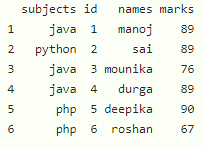### Example 1: Summarize One Variable & Group by One Variable

Here, we are going to get the summary of one variable by grouping it with one variable.

Syntax:

`aggregate(sum_column ~ group_column, data, FUN=sum)`

In this example, We are going to use the sum function to get some of marks by grouping with subjects.

## R

 `# create the dataframe with 4 columns ` `data = ``data.frame``(subjects=``c``(``"java"``, ``"python"``, ``"java"``, ` `                             ``"java"``, ``"php"``, ``"php"``), ` `                  ``id=``c``(1, 2, 3, 4, 5, 6), ` `                  ``names=``c``(``"manoj"``, ``"sai"``, ``"mounika"``, ` `                          ``"durga"``, ``"deepika"``, ``"roshan"``), ` `                  ``marks=``c``(89, 89, 76, 89, 90, 67)) ` ` `  `# get sum of marks  by grouping with subjects ` `aggregate``(marks~ subjects, data, FUN=sum) `

Output: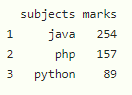### Example 2: Summarize One Variable & Group by Multiple Variables

Here we are going to get the summary of one variable by grouping it with one or more variables. We have to use the + operator to group multiple columns.

Syntax:

aggregate(sum_column ~ group_column1+group_column2+……………group_columnn, data, FUN=sum)

In this example, We are going to group names and subjects to get sum of marks.

## R

 `# create the dataframe with 4 columns ` `data = ``data.frame``(subjects=``c``(``"java"``, ``"python"``, ``"java"``, ` `                             ``"java"``, ``"php"``, ``"php"``), ` `                  ``id=``c``(1, 2, 3, 4, 5, 6), ` `                  ``names=``c``(``"manoj"``, ``"sai"``, ``"mounika"``, ` `                          ``"durga"``, ``"deepika"``, ``"roshan"``), ` `                  ``marks=``c``(89, 89, 76, 89, 90, 67)) ` ` `  `# get sum of marks  by grouping with subjects and names ` `aggregate``(marks~ subjects+names, data, FUN=sum) `

Output: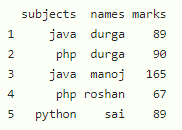### Example 3: Summarize Multiple Variables & Group by One Variable

Here we are going to get the summary of one or more variables by grouping with one variable. We will use cbind() function known as column binding to get a summary of multiple variables.

Syntax:

aggregate(cbind(sum_column1,sum_column2,.,sum_column n) ~ group_column1+group_column2+……………group_columnn, data, FUN=sum)

In this example, We are going to get sum of marks and id by grouping with subjects.

## R

 `# create the dataframe with 4 columns ` `data = ``data.frame``(subjects=``c``(``"java"``, ``"python"``, ``"java"``,  ` `                             ``"java"``, ``"php"``, ``"php"``), ` `                  ``id=``c``(1, 2, 3, 4, 5, 6), ` `                  ``names=``c``(``"manoj"``, ``"sai"``, ``"mounika"``, ` `                          ``"durga"``, ``"deepika"``, ``"roshan"``), ` `                  ``marks=``c``(89, 89, 76, 89, 90, 67)) ` ` `  `# get sum of marks and id by grouping with subjects ` `aggregate``(``cbind``(marks, id)~ subjects, data, FUN=sum) `

Output: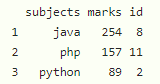### Example 4: Summarize Multiple Variables & Group by Multiple Variables

Here, we are going to get the summary of one or more variables by grouping them with one or more variables. We can use cbind() for combining one or more variables and the ‘+’ operator for grouping multiple variables.

Syntax:

aggregate(cbind(sum_column1,.,sum_column n)~ group_column1+….+group_column n, data, FUN=sum)

In this example, We are going to get sum of marks and id by grouping them with subjects and names.

## R

 `# create the dataframe with 4 columns ` `data = ``data.frame``(subjects=``c``(``"java"``, ``"python"``, ``"java"``, ` `                             ``"java"``, ``"php"``, ``"php"``), ` `                  ``id=``c``(1, 2, 3, 4, 5, 6), ` `                  ``names=``c``(``"manoj"``, ``"sai"``, ``"mounika"``, ` `                          ``"durga"``, ``"deepika"``, ``"roshan"``), ` `                  ``marks=``c``(89, 89, 76, 89, 90, 67)) ` ` `  `# get sum of marks and id by grouping  ` `# with subjects and names ` `aggregate``(``cbind``(marks, id)~ subjects+names, data, FUN=sum) `

Output: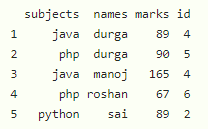My Personal Notes arrow_drop_up
Related Articles